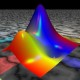Ask Your Question

# Rotation of a curve

Here is a box with a curve in it

ppplot=polygon([( 0 , 0 ), ( 0 , 10 ), ( 1 , 10 ),( 1 , 0 )], color = "#bbd2e1")
ppplot+=plot(1/x,(x, 0.1, 1), color="red")
ppplot+=plot(-10*x+10,(x, 0, 1), color="green",linestyle="--")
ppplot+=line([(0, 10), (1,10),(1,0)], color="green",linestyle="--")
ppplot+=circle((1,10), .1,edgecolor="green",fill=true, facecolor="green")
show(ppplot,aspect_ratio=0.05)


I have two questions :

1) how to impeach that the aspect-ratio be applied to circle()

2) how to obtain a new curve where $1/x$ but this time with the origin of the axes in the green dot (symetry by the dashed line.

3) In the same way how to change the normal cartesian representation of a function from (x,y) to (y,x) in plot

edit retag close merge delete

## 1 Answer

Sort by » oldest newest most voted1) To my knowledge, you can't, since the aspect_ratio option in show has a global effect and overrides any previous setting which can be done. Just draw an ellipse with the right size.

2) It is not clear to me what you mean. Do you want the curve which is strictly symmetric to $y=1/x$ with respect to $y=-10x+10$? Or do you want to plot a curve with the shape of $y=1/x$ but translated to $(1,10)$ and the directions of the axes reversed ($x$-axis pointing to the left, $y$-axis pointing down)? They are two different things. The code below does the second one.

3) The $x$-coordinate is the horizontal one, the $y$-coordinate is the vertical one. Given a function $f$, we usually consider that $x$ is the independent variable and that $y$ is the dependent one. So I interpret your question as follows: how to plot a function $f$ when the independent variable is $y$. If I am right, the answer could be: don't use plot, use parametric_plot or implicit_plot. More specifically, to draw $x=f(y)$, with $y\in[c,d]$, use

parametric_plot((f(y),y), (y,c,d))


or

implicit_plot(x==f(y), (x,a,b), (y,c,d))


for suitable values of $a$ and $b$.

Check this code, which modifies yours:

var("x,y")
x1, x2, y1, y2 = 0, 1, 0, 10
p = polygon([(x1,y1), (x1,y2), (x2,y2), (x2,y1)], color="#bbd2e1")
p += plot(-10*x+10, (x,x1,x2), color="green", linestyle="dashed")

f(x) = 1/x
p += plot(f(x), (x,x1+0.1,x2), color="red")
x0, y0 = 1, 10
p += implicit_plot(f(x0-x)==y0-y, (x,x0-x2,x0-x1), (y,y0-y2,y0-y1), color="yellow")

scale = 0.05
radius = 0.02
p += ellipse((x2,y2), radius, radius/scale, edgecolor="green",
fill=true, facecolor="green")
show(p, aspect_ratio=scale)


This is the result:more

## Your Answer

Please start posting anonymously - your entry will be published after you log in or create a new account.

Add Answer

## Stats

Asked: 2020-05-29 11:36:35 +0200

Seen: 59 times

Last updated: May 29 '20